# 准确率（Accuracy）

$Accuracy = \frac{TP+TN}{TP+TN+FP+FN}$

• 真正例(True Positive, TP)：被模型预测为正的正样本；
• 假正例(False Positive, FP)：被模型预测为正的负样本；
• 假负例(False Negative, FN)：被模型预测为负的正样本；
• 真负例(True Negative, TN)：被模型预测为负的负样本；

# 精确率（Precision）、召回率（Recall）

$Precision = \frac{TP}{TP+FP}$

$Recall = \frac{TP}{TP+FN}$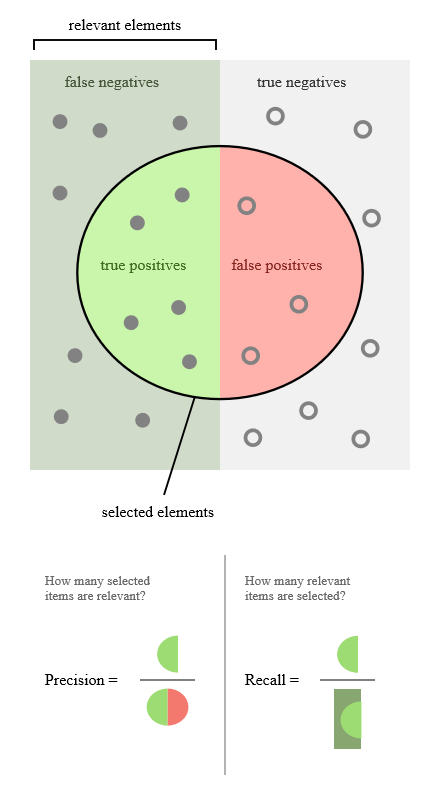# P-R曲线

P-R曲线（Precision Recall Curve）正是描述精确率/召回率变化的曲线，P-R曲线定义如下：根据学习器的预测结果（一般为一个实值或概率）对测试样本进行排序，将最可能是“正例”的样本排在前面，最不可能是“正例”的排在后面，按此顺序逐个把样本作为“正例”进行预测，每次计算出当前的P值和R值，如下图所示：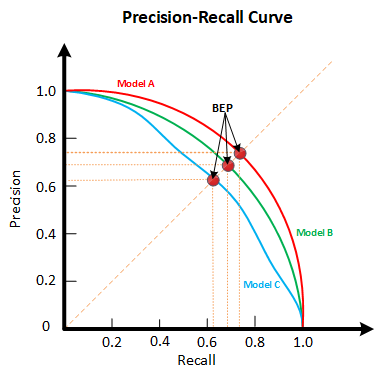P-R曲线如何评估呢？若一个学习器A的P-R曲线被另一个学习器B的P-R曲线完全包住，则称：B的性能优于A。若A和B的曲线发生了交叉，则谁的曲线下的面积大，谁的性能更优。但一般来说，曲线下的面积是很难进行估算的，所以衍生出了“平衡点”（Break-Event Point，简称BEP），即当P=R时的取值，平衡点的取值越高，性能更优。

# F1-Score

$\frac{1}{F_{\beta}}=\frac{1}{1+\beta^{2}} \cdot\left(\frac{1}{P}+\frac{\beta^{2}}{R}\right)$

$F_{\beta}=\frac{\left(1+\beta^{2}\right) \times P \times R}{\left(\beta^{2} \times P\right)+R}$

$\frac{1}{F 1}=\frac{1}{2} \cdot\left(\frac{1}{P}+\frac{1}{R}\right)$

$F1=\frac{2 \times P \times R}{P+R} = \frac{2 \times TP}{样例总数+TP-TN}$

# ROC曲线

ROC以及后面要讲到的AUC，是分类任务中非常常用的评价指标，本文将详细阐述。可能有人会有疑问，既然已经这么多评价标准，为什么还要使用ROC和AUC呢？

• 真正率(True Positive Rate , TPR)，又称灵敏度：

$TPR = \frac{正样本预测正确数}{正样本总数} = \frac{TP}{TP+FN}$

​ 其实我们可以发现灵敏度和召回率是一模一样的，只是名字换了而已

• 假负率(False Negative Rate , FNR) ：

$FNR = \frac{正样本预测错误数}{正样本总数} = \frac{FN}{TP+FN}$

• 假正率(False Positive Rate , FPR) ：

$FPR = \frac{负样本预测错误数}{负样本总数} = \frac{FP}{TN+FP}$

• 真负率(True Negative Rate , TNR)，又称特异度：

$TNR = \frac{负样本预测正确数}{负样本总数} = \frac{TN}{TN+FP}$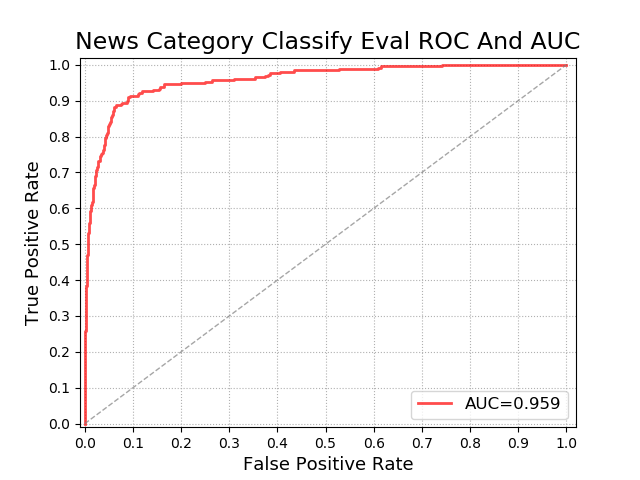## 阈值问题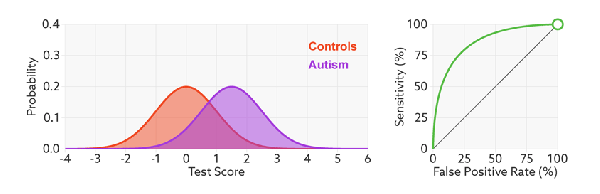## 判断模型性能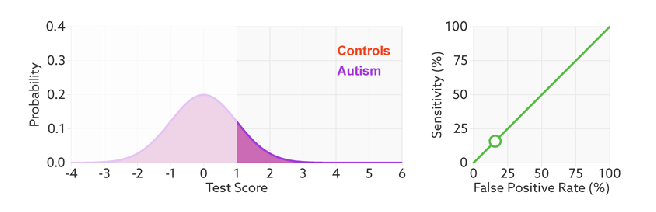## 无视样本不平衡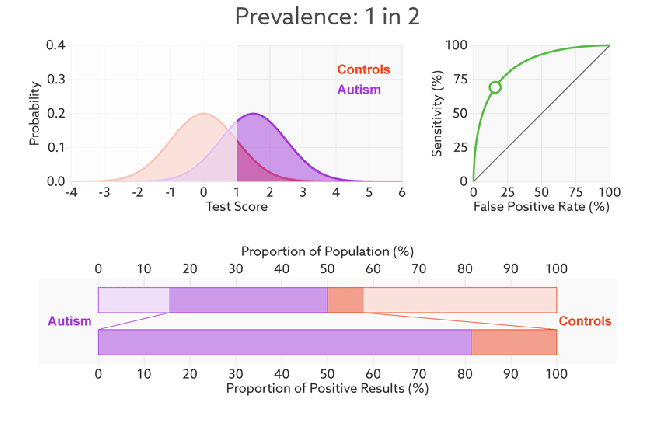# AUC

AUC(Area Under Curve)又称为曲线下面积，是处于ROC Curve下方的那部分面积的大小。上文中我们已经提到，对于ROC曲线下方面积越大表明模型性能越好，于是AUC就是由此产生的评价指标。通常，AUC的值介于0.5到1.0之间，较大的AUC代表了较好的Performance。如果模型是完美的，那么它的AUC = 1，证明所有正例排在了负例的前面，如果模型是个简单的二类随机猜测模型，那么它的AUC = 0.5，如果一个模型好于另一个，则它的曲线下方面积相对较大，对应的AUC值也会较大。

## 物理意义

AUC对所有可能的分类阈值的效果进行综合衡量。首先AUC值是一个概率值，可以理解为随机挑选一个正样本以及一个负样本，分类器判定正样本分值高于负样本分值的概率就是AUC值。简言之，AUC值越大，当前的分类算法越有可能将正样本分值高于负样本分值，即能够更好的分类。

# 混淆矩阵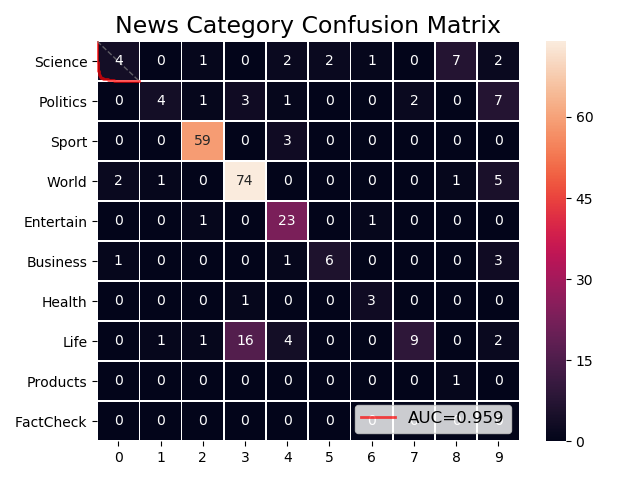# 多分类问题

$\operatorname{macro}P=\frac{1}{n} \sum_{i=1}^{n} P_{i}$

$\operatorname{macro}R=\frac{1}{n} \sum_{i=1}^{n} R_{i}$

$\operatorname{macro} F 1=\frac{2 \times \operatorname{macro} P \times \operatorname{macro} R}{\operatorname{macro} P+\operatorname{macro}R}$

$\operatorname{micro} P=\frac{\overline{T P}}{\overline{T P}+\overline{F P}}$

$\operatorname{micro}R=\frac{\overline{T P}}{\overline{T P}+\overline{F N}}$

$\operatorname{micro} F 1=\frac{2 \times \operatorname{micro} P \times \operatorname{micro} R}{\operatorname{micro} P+\operatorname{micro}R}$

posted @ 2019-08-27 14:52  郭耀华  阅读(...)  评论(...编辑  收藏